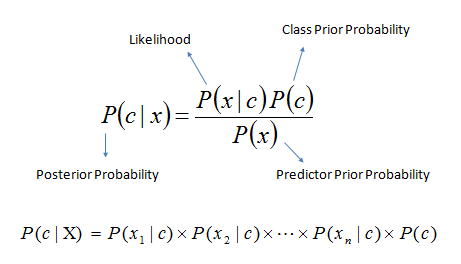## Friday, 6 November 2015

### Using Naive Bayes classifier

This is most basic classifier, It uses concept of probability in order to predict the class of input feature data . Read More

We gonna see how to use Naive Bayes Classifier very easily using python.Bayes rule

### Prerequisite:

• Python 2
• sklearn [Python Module]
• Numpy  [Python Module]

We are going to apply this algorithm on Iris dataset .

Iris dataset : There are total 150 training set in this dataset. and each training set consist of  4 feature and corresponding class ( There 3 class in Iris dataset. represented by 0 for setosa ,1 for versicolor ,2 for virginica ) .
These 4 feature used to classify data in 3 classes of flowers .Read More

We are going to use sklearn python package, we use inbuilt function in sklearn for naive bayes classifier. Fist train the our classifier then predict class for your input, then calculate accuracy of result.
Fallow code comments for better understanding.

## Naive Bayes Classifier [ Python ] [Github Link]

1. __auther__='www.codecops.in'
2. # Classify Iris data Using Naive Bayes
3. #An Example Of Supervised Learning
5. from sklearn.naive_bayes import GaussianNB
6. import numpy as np
7. #{{ Getting Iris Dataset
9. X=data.data #input Features
10. Y=data.target #Class of corresponding Input
11. #}}
12. if __name__=='__main__':
13.     # clsf == classifire :)
14.     clsf=GaussianNB()
15.     #{{training our classifire using Naive_bayes
16.     #!for IRis data Classification
17.     clsf.fit(X,Y)
18.     #}}

19.     # Test fot All data
20.     print '''  _______Input_______\
21.            __Real Class__\
22.            Prediction class '''
23.     pred=[]
24.     for i in range(len(X)):
25.         pred.append(clsf.predict(X[i])) #storing Pred result
26.         print X[i],'\t\t\t',Y[i],'\t\t\t',pred[-1]
27.     from sklearn.metrics import accuracy_score
28.     print "Accuracy Test Using accuracy_score function"
29.     pred=np.array(pred)
30.     print accuracy_score(pred,Y)*100,'%  Wow _/\_ Not Bad'

Output :Naive Bayes classifire accuracy on Iris data